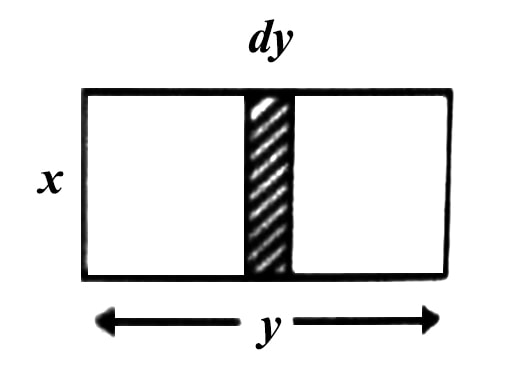# Application of Integration in Some Cases

Application of integration in some cases:

(i) General integration of vector quantity is similar to scalar quantity. Let the vector A(t) = ȋ Ax(t) + ĵ Ay(t) + ƙ Az (t) be integral of a scalar variable t, then;

∫ A(t) dt = ȋ ∫ Ax (t) dt + ĵ ∫ Ay (t) dt + ƙ ∫ Az (t) dt.

It is called the indefinite integral of A (t).

(ii) In many cases integration is opposite to differentiation: for example-

∫ cos x dx = sin x

d/dx (sin x) = cos x

i.e., if sin x is differentiated we get cos x and if cos x is integrated we get sin x.(iii) If a rectangle consists of innumerable elements of length x and width dy [Figure] then area of the rectangle will be, ∫ xdy = x∫ dy = xy

(iv) If limit of width is mentioned, then area = y0 x dy = x [y]y0 = x (y-0) = xy.